首页 > 酒店 > 北京> 北京王府井银地宾馆

### 北京王府井银地宾馆296

3.8分 /（945人评价）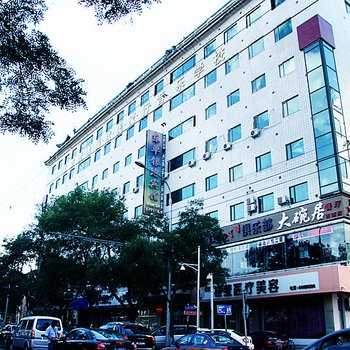•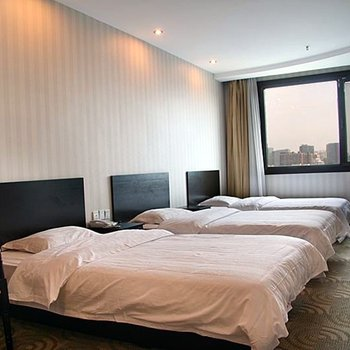•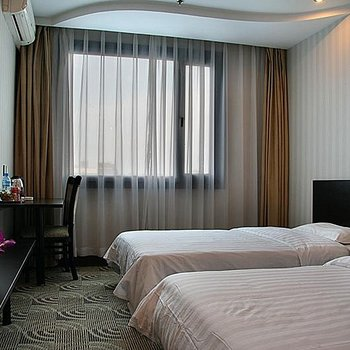•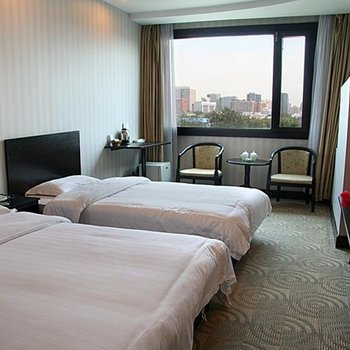•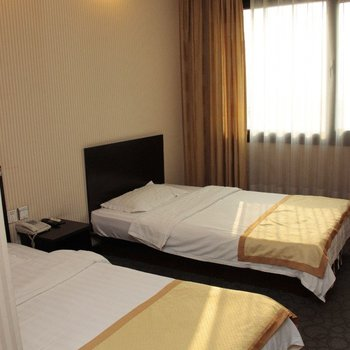•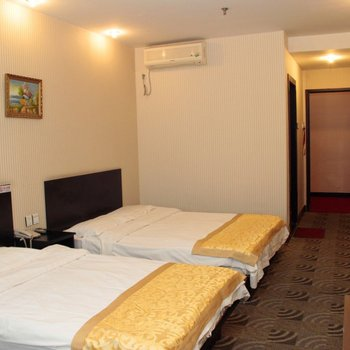•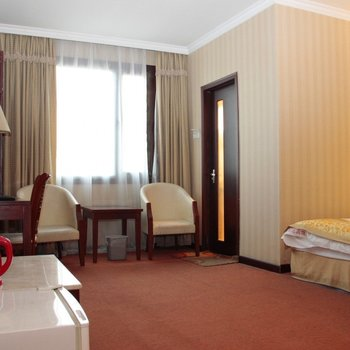•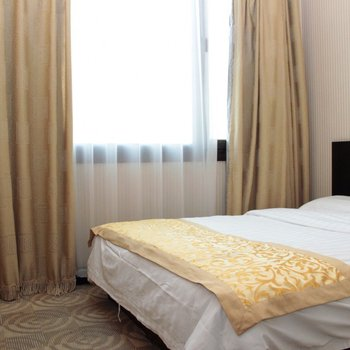•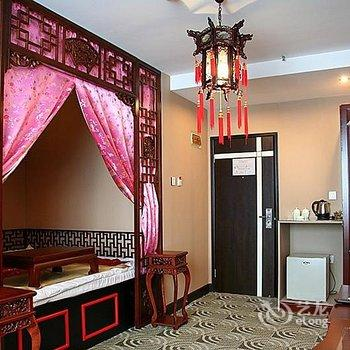•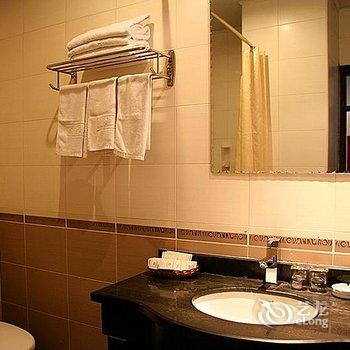•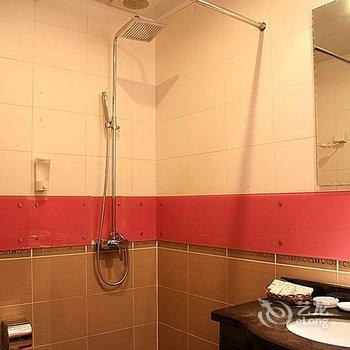••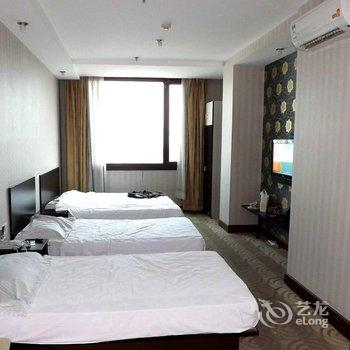•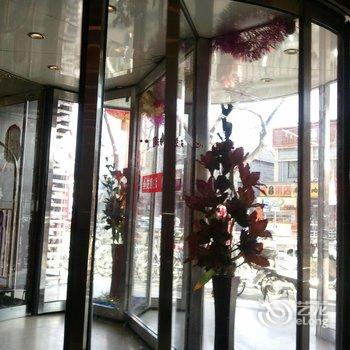••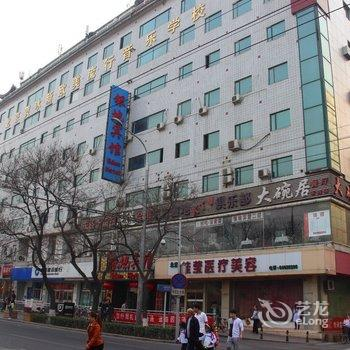•••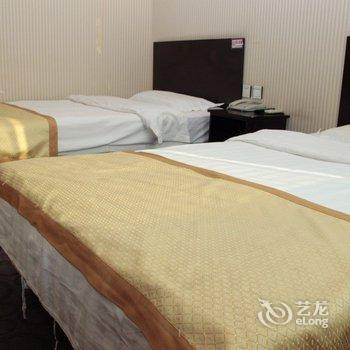•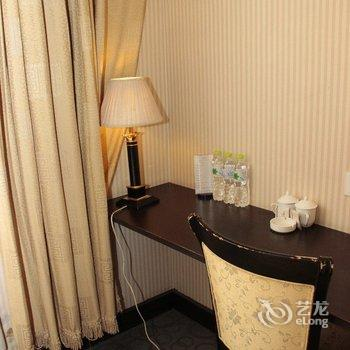•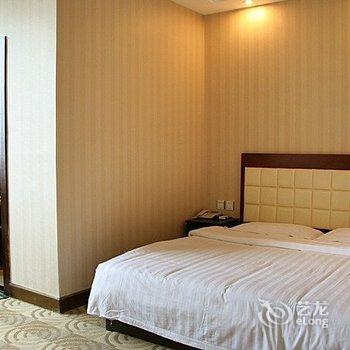•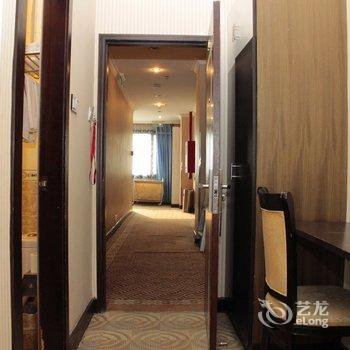•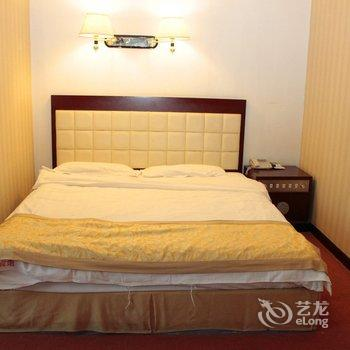•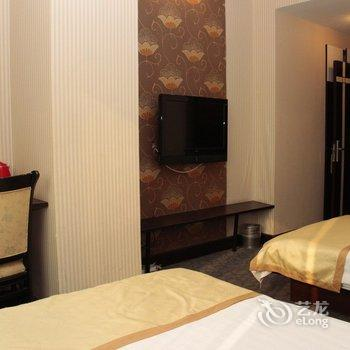•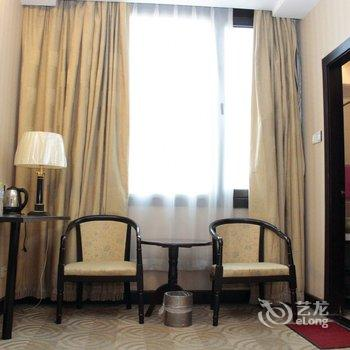•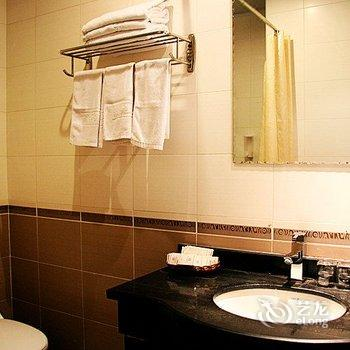•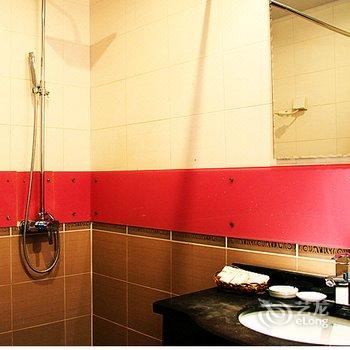•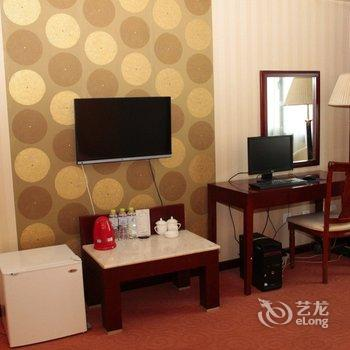•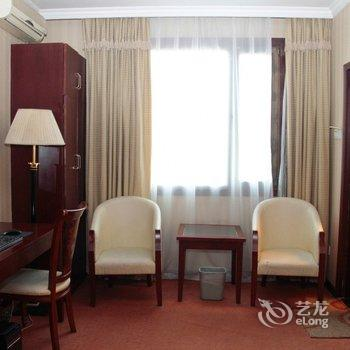•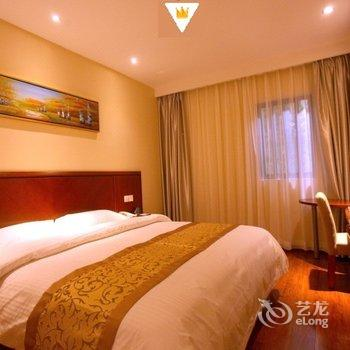•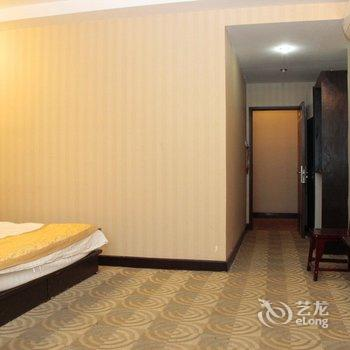•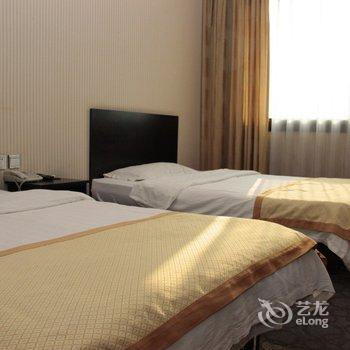•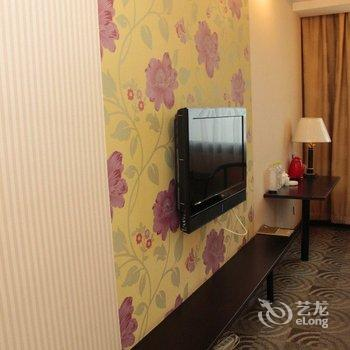•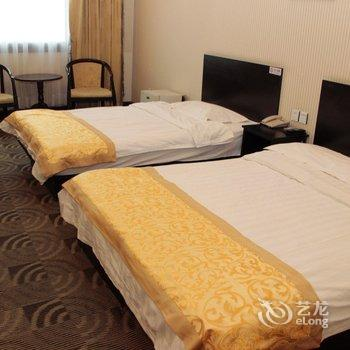•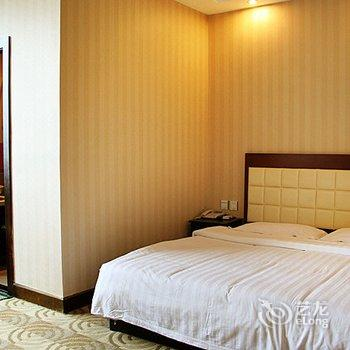•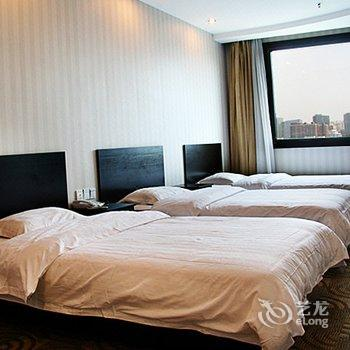••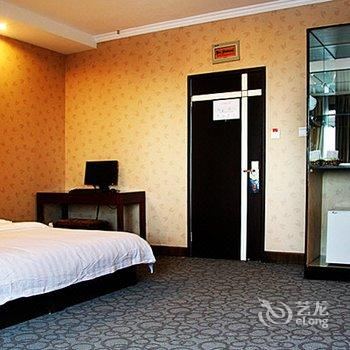•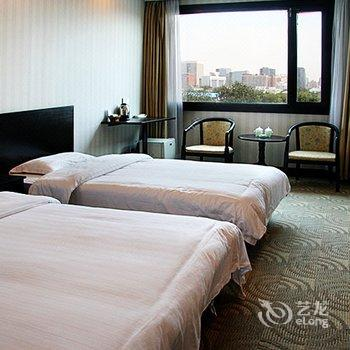•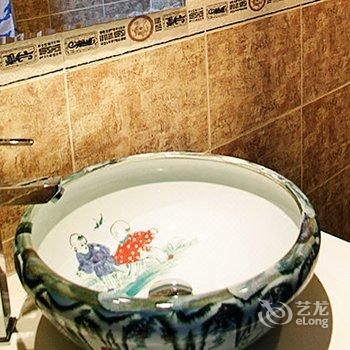•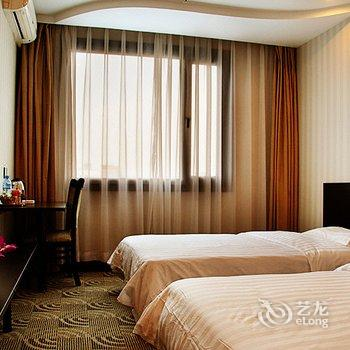•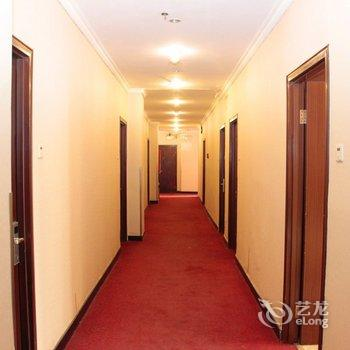•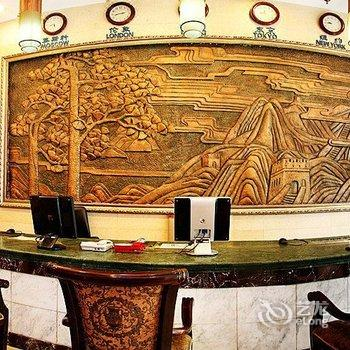••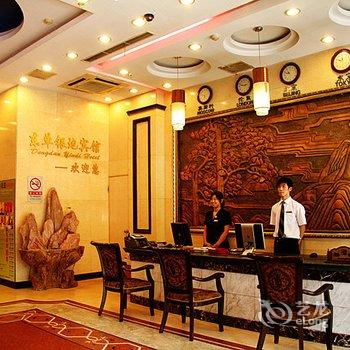•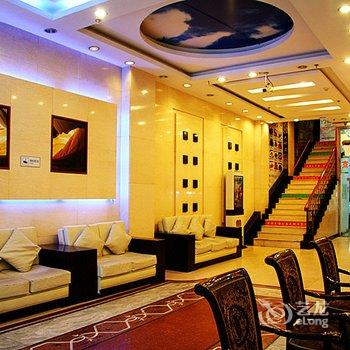•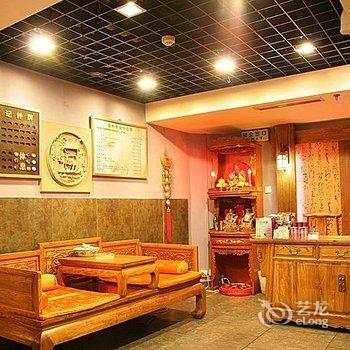•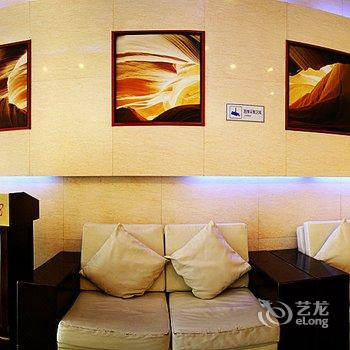•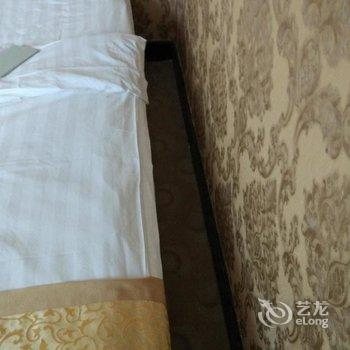•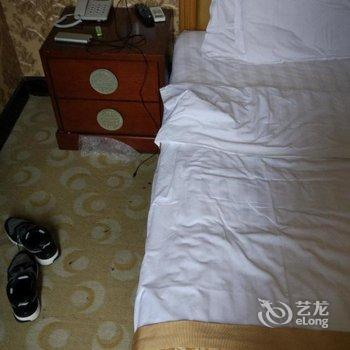•••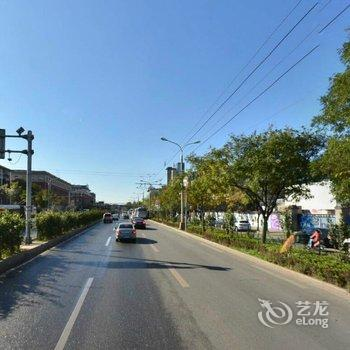••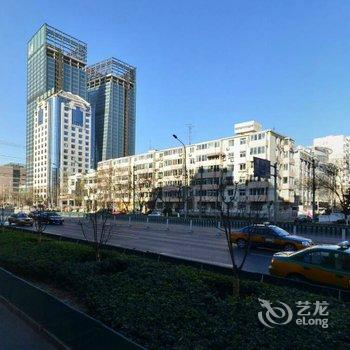•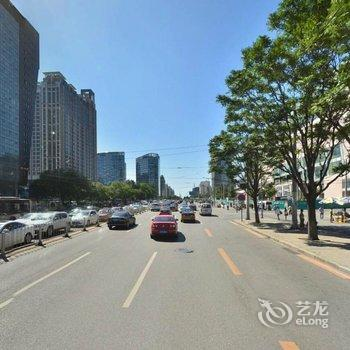•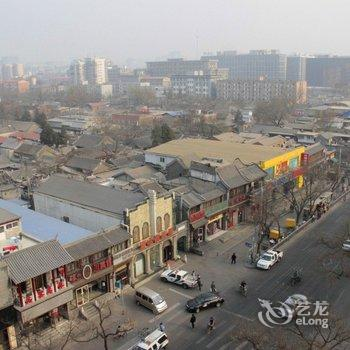••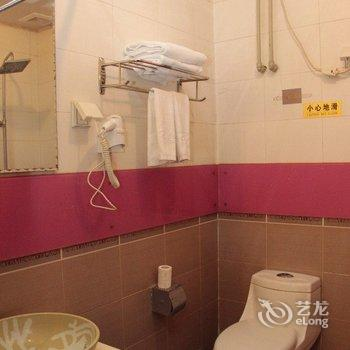•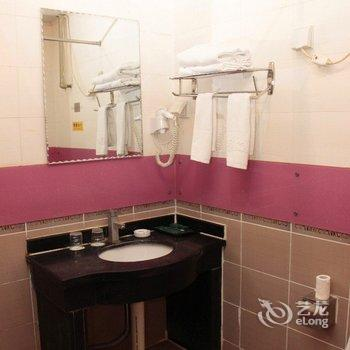•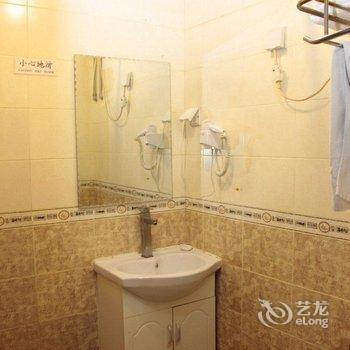•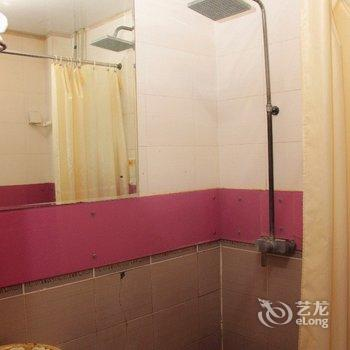•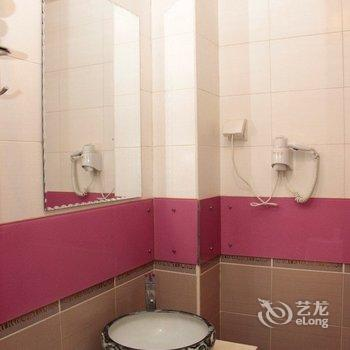•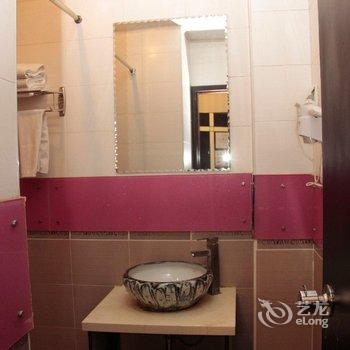•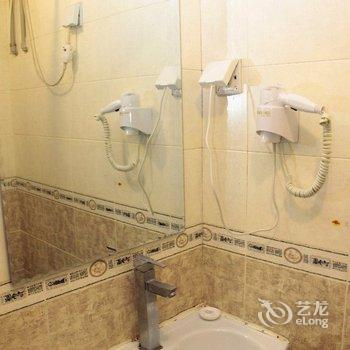•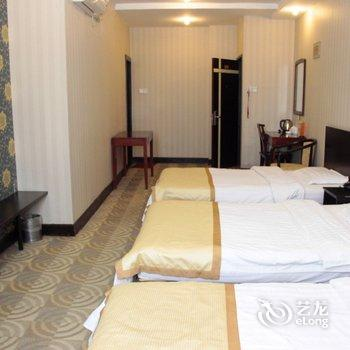•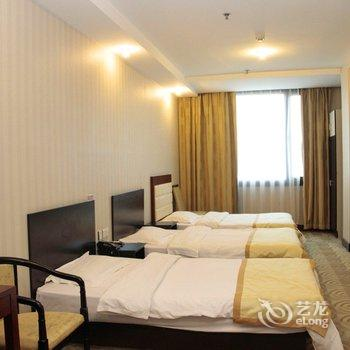•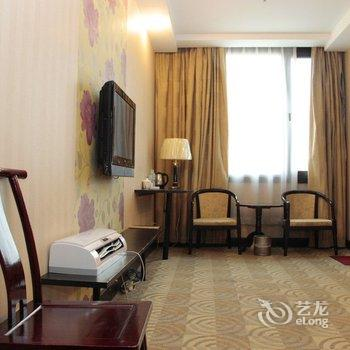•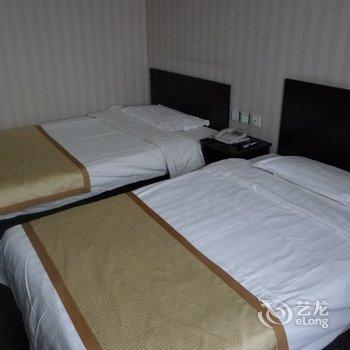•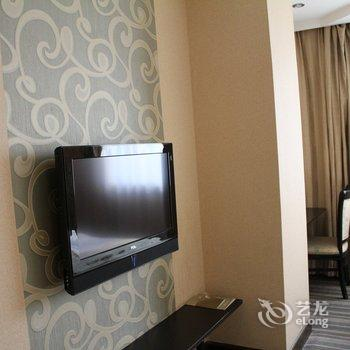•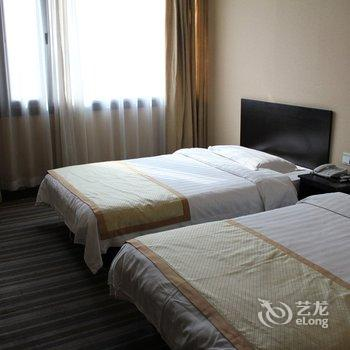••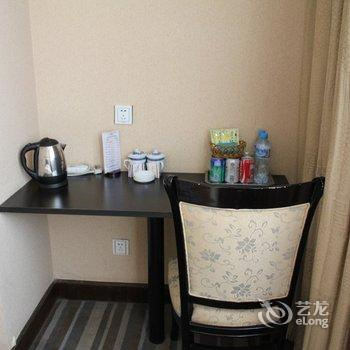•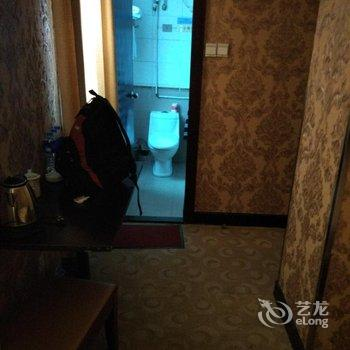•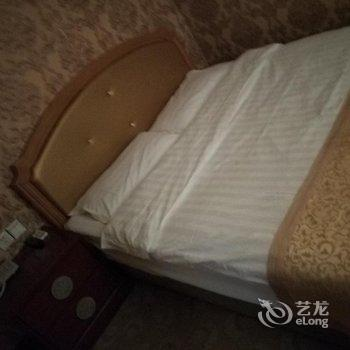•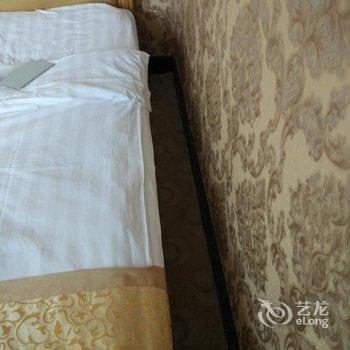•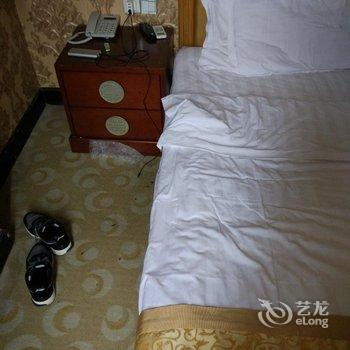#### 普通大床间

• 不含早（预付）
• 大床1.5米
• 有窗
• 免费无线
• ￥296
• 有房
• 预付
• 预订
• 标准价
• 大床1.5米
• 有窗
• 免费无线
• ￥296
• 有房
• 预付
• 预订
• 标准大床间(无早)
• 大床1.5米
• 有窗
• 免费无线
• ￥298
• 有房
• 预付
• 预订
• 标准大床间(无早)
• 大床1.5米
• 有窗
• 免费无线
• ￥298
• 有房
• 预付
• 预订
• 标准大床间(提前1天预订)[无早]
• 大床1.5米
• 有窗
• 免费无线
• ￥298
• 有房
• 预付
• 预订
• 标准大床间(无早)(今日特价)
• 大床1.5米
• 有窗
• 免费无线
• ￥298
• 有房
• 预付
• 预订
• 标准大床间(限时促销)(无早)(大床)
• 大床1.5米
• 有窗
• 免费无线
• ￥298
• 有房
• 预付
• 预订
• 标准大床间(限时 特惠)(无早)(大床)
• 大床1.5米
• 有窗
• 免费无线
• ￥298
• 有房
• 预付
• 预订
• 标准价
• 大床1.5米
• 有窗
• 免费无线
• ￥298
• 有房
• 预付
• 预订
• (今日特价)
• 大床1.5米
• 有窗
• 免费无线
• ￥298
• 有房
• 预付
• 预订
• 标准价
• 大床1.5米
• 有窗
• 免费无线
• ￥298
• 有房
• 预付
• 预订
• (今日特价)
• 大床1.5米
• 有窗
• 免费无线
• ￥298
• 有房
• 预付
• 预订
• 标准价
• 大床1.5米
• 有窗
• 免费无线
• ￥298
• 有房
• 预付
• 预订
• (大床)(限时 特惠)
• 大床1.5米
• 有窗
• 免费无线
• ￥298
• 有房
• 预付
• 预订
• (大床)(限时促销)
• 大床1.5米
• 有窗
• 免费无线
• ￥298
• 有房
• 预付
• 预订
• (大床)(提前1天预订)(今日特价)
• 大床1.5米
• 有窗
• 免费无线
• ￥298
• 有房
• 预付
• 预订
• (大床)(今日特价)
• 大床1.5米
• 有窗
• 免费无线
• ￥298
• 有房
• 预付
• 预订
• (大床)(今日特价)
• 大床1.5米
• 有窗
• 免费无线
• ￥298
• 有房
• 预付
• 预订
• (今日特价)
• 大床1.5米
• 有窗
• 免费无线
• ￥298
• 有房
• 预付
• 预订
• (今日特价)
• 大床1.5米
• 有窗
• 免费无线
• ￥298
• 有房
• 预付
• 预订
• (提前1天预订)
• 大床1.5米
• 有窗
• 免费无线
• ￥298
• 有房
• 预付
• 预订
• 标准价
• 大床1.5米
• 有窗
• 免费无线
• ￥308
• 有房
• 预付
• 预订
• (大床)
• 大床1.5米
• 有窗
• 免费无线
• ￥313
• 有房
• 预付
• 预订
• (大床)
• 大床1.5米
• 有窗
• 免费无线
• ￥314
• 有房
• 预付
• 预订
• 标准价
• 大床1.5米
• 有窗
• 免费无线
• ￥314
• 有房
• 预付
• 预订
• (限量抢购)
• 大床1.5米
• 有窗
• 免费无线
• ￥314
• 有房
• 预付
• 预订
• 标准价
• 大床1.5米
• 有窗
• 免费无线
• ￥315
• 有房
• 到店付
• 预订
• 标准价
• 大床1.5米
• 有窗
• 免费无线
• ￥319
• 有房
• 预付
• 预订
• (大床)
• 大床1.5米
• 有窗
• 免费无线
• ￥319
• 有房
• 预付
• 预订
• 标准价
• 大床1.5米
• 有窗
• 免费无线
• ￥319
• 有房
• 预付
• 预订
• 标准价
• 大床1.5米
• 有窗
• 免费无线
• ￥319
• 有房
• 预付
• 预订
• 标准价
• 大床1.5米
• 有窗
• 免费无线
• ￥319
• 有房
• 预付
• 预订
• 标准价
• 大床1.5米
• 有窗
• 免费无线
• ￥319
• 有房
• 预付
• 预订
• 标准价
• 大床1.5米
• 有窗
• 免费无线
• ￥319
• 有房
• 预付
• 预订
• 标准价
• 大床1.5米
• 有窗
• 免费无线
• ￥319
• 有房
• 预付
• 预订
• 标准价
• 大床1.5米
• 有窗
• 免费无线
• ￥323
• 有房
• 预付
• 预订
• (限量特惠)
• 大床1.5米
• 有窗
• 免费无线
• ￥326
• 有房
• 预付
• 预订
• 不含早（预付）
• 大床1.5米
• 有窗
• 免费无线
• ￥331
• 有房
• 预付
• 预订
• 标准价
• 大床1.5米
• 有窗
• 免费无线
• ￥331
• 有房
• 预付
• 预订
• (提前1天预订)
• 大床1.5米
• 有窗
• 免费无线
• ￥331
• 有房
• 预付
• 预订
• (特价大促销)
• 大床1.5米
• 有窗
• 免费无线
• ￥331
• 有房
• 预付
• 预订
• 标准价
• 大床1.5米
• 有窗
• 免费无线
• ￥337
• 有房
• 预付
• 预订
• 标准价
• 大床1.5米
• 有窗
• 免费无线
• ￥348
• 有房
• 预付
• 预订
• (大床)
• 大床1.5米
• 有窗
• 免费无线
• ￥361
• 有房
• 预付
• 预订
• (今日特价)
• 大床1.5米
• 有窗
• 免费无线
• ￥362
• 有房
• 预付
• 预订
• 标准大床间(度假)(今日特价 )
• 大床1.5米
• 有窗
• 免费无线
• ￥298
• 满房
• 预付
• 满房
• 标准大床间(双人入住)(无早)(今日特价 )(大床)
• 大床1.5米
• 有窗
• 免费无线
• ￥298
• 满房
• 预付
• 满房
• 标准大床间(提前1天预订)(无早)(今日特价 )(大床)
• 大床1.5米
• 有窗
• 免费无线
• ￥298
• 满房
• 预付
• 满房
• 标准大床间(无早)(今日特价 )
• 大床1.5米
• 有窗
• 免费无线
• ￥298
• 满房
• 预付
• 满房
• 标准大床间(无早)(今日特价 )(普通,金牌,白金,钻石会员 特惠)
• 大床1.5米
• 有窗
• 免费无线
• ￥298
• 满房
• 预付
• 满房
• 标准大床间(无早)(今日特价 )(大床)
• 大床1.5米
• 有窗
• 免费无线
• ￥298
• 满房
• 预付
• 满房
• (特惠)
• 大床1.5米
• 有窗
• 免费无线
• ￥312
• 满房
• 预付
• 满房
• (2间起订)
• 大床1.5米
• 有窗
• 免费无线
• ￥312
• 满房
• 预付
• 满房
• (大床)(特惠)
• 大床1.5米
• 有窗
• 免费无线
• ￥312
• 满房
• 预付
• 满房
• 标准价
• 大床1.5米
• 有窗
• 免费无线
• ￥326
• 满房
• 预付
• 满房
• (大床)
• 大床1.5米
• 有窗
• 免费无线
• ￥326
• 满房
• 预付
• 满房
• 标准价
• 大床1.5米
• 有窗
• 免费无线
• ￥326
• 满房
• 预付
• 满房
• (特价)
• 大床1.5米
• 有窗
• 免费无线
• ￥349
• 满房
• 预付
• 满房
• (今日特价)
• 大床1.5米
• 有窗
• 免费无线
• ￥362
• 满房
• 预付
• 满房
• (大床)
• 大床1.5米
• 有窗
• 免费无线
• ￥372
• 满房
• 预付
• 满房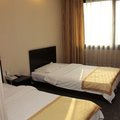#### 普通双床间

• 不含早（预付）
• 双床1.2米
• 有窗
• 免费无线
• ￥296
• 有房
• 预付
• 预订
• 标准双床间(特惠)(无早)
• 双床1.2米
• 有窗
• 免费无线
• ￥298
• 有房
• 预付
• 预订
• 标准双床间(无早)
• 双床1.2米
• 有窗
• 免费无线
• ￥298
• 有房
• 预付
• 预订
• 标准双床间(提前1天预订)[无早]
• 双床1.2米
• 有窗
• 免费无线
• ￥298
• 有房
• 预付
• 预订
• 标准双床间(无早)
• 双床1.2米
• 有窗
• 免费无线
• ￥298
• 有房
• 预付
• 预订
• 标准双床间(特惠)(无早)(双床)
• 双床1.2米
• 有窗
• 免费无线
• ￥298
• 有房
• 预付
• 预订
• 标准双床间(无早)(特价大促销)
• 双床1.2米
• 有窗
• 免费无线
• ￥298
• 有房
• 预付
• 预订
• 标准价
• 双床1.2米
• 有窗
• 免费无线
• ￥298
• 有房
• 预付
• 预订
• (今日特价)
• 双床1.2米
• 有窗
• 免费无线
• ￥298
• 有房
• 预付
• 预订
• 标准价
• 双床1.2米
• 有窗
• 免费无线
• ￥298
• 有房
• 预付
• 预订
• (特惠)
• 双床1.2米
• 有窗
• 免费无线
• ￥298
• 有房
• 预付
• 预订
• (双床)(特惠)
• 双床1.2米
• 有窗
• 免费无线
• ￥298
• 有房
• 预付
• 预订
• (特价大促销)
• 双床1.2米
• 有窗
• 免费无线
• ￥298
• 有房
• 预付
• 预订
• (双床)(提前1天预订)(今日特价)
• 双床1.2米
• 有窗
• 免费无线
• ￥298
• 有房
• 预付
• 预订
• (提前1天预订)
• 双床1.2米
• 有窗
• 免费无线
• ￥298
• 有房
• 预付
• 预订
• (双床)(今日特价)
• 双床1.2米
• 有窗
• 免费无线
• ￥298
• 有房
• 预付
• 预订
• (双床)(特惠专享)
• 双床1.2米
• 有窗
• 免费无线
• ￥298
• 有房
• 预付
• 预订
• (今日特价)
• 双床1.2米
• 有窗
• 免费无线
• ￥298
• 有房
• 预付
• 预订
• (今日特价)
• 双床1.2米
• 有窗
• 免费无线
• ￥298
• 有房
• 预付
• 预订
• 标准价
• 双床1.2米
• 有窗
• 免费无线
• ￥298
• 有房
• 预付
• 预订
• 标准价
• 双床1.2米
• 有窗
• 免费无线
• ￥308
• 有房
• 预付
• 预订
• (双床)
• 双床1.2米
• 有窗
• 免费无线
• ￥313
• 有房
• 预付
• 预订
• (双床)
• 双床1.2米
• 有窗
• 免费无线
• ￥314
• 有房
• 预付
• 预订
• 标准价
• 双床1.2米
• 有窗
• 免费无线
• ￥314
• 有房
• 预付
• 预订
• (特惠专享)
• 双床1.2米
• 有窗
• 免费无线
• ￥314
• 有房
• 预付
• 预订
• 标准价
• 双床1.2米
• 有窗
• 免费无线
• ￥315
• 有房
• 到店付
• 预订
• 标准价
• 双床1.2米
• 有窗
• 免费无线
• ￥319
• 有房
• 预付
• 预订
• (双床)
• 双床1.2米
• 有窗
• 免费无线
• ￥319
• 有房
• 预付
• 预订
• 标准价
• 双床1.2米
• 有窗
• 免费无线
• ￥319
• 有房
• 预付
• 预订
• 标准价
• 双床1.2米
• 有窗
• 免费无线
• ￥319
• 有房
• 预付
• 预订
• 标准价
• 双床1.2米
• 有窗
• 免费无线
• ￥319
• 有房
• 预付
• 预订
• 标准价
• 双床1.2米
• 有窗
• 免费无线
• ￥319
• 有房
• 预付
• 预订
• 标准价
• 双床1.2米
• 有窗
• 免费无线
• ￥319
• 有房
• 预付
• 预订
• 标准价
• 双床1.2米
• 有窗
• 免费无线
• ￥319
• 有房
• 预付
• 预订
• 标准价
• 双床1.2米
• 有窗
• 免费无线
• ￥319
• 有房
• 预付
• 预订
• 标准价
• 双床1.2米
• 有窗
• 免费无线
• ￥323
• 有房
• 预付
• 预订
• (限量特惠)
• 双床1.2米
• 有窗
• 免费无线
• ￥326
• 有房
• 预付
• 预订
• 不含早（预付）
• 双床1.2米
• 有窗
• 免费无线
• ￥331
• 有房
• 预付
• 预订
• 标准价
• 双床1.2米
• 有窗
• 免费无线
• ￥331
• 有房
• 预付
• 预订
• (提前1天预订)
• 双床1.2米
• 有窗
• 免费无线
• ￥331
• 有房
• 预付
• 预订
• (特价大促销)
• 双床1.2米
• 有窗
• 免费无线
• ￥331
• 有房
• 预付
• 预订
• 标准价
• 双床1.2米
• 有窗
• 免费无线
• ￥337
• 有房
• 预付
• 预订
• 标准价
• 双床1.2米
• 有窗
• 免费无线
• ￥348
• 有房
• 预付
• 预订
• (特惠房)
• 双床1.2米
• 有窗
• 免费无线
• ￥349
• 有房
• 预付
• 预订
• (双床)
• 双床1.2米
• 有窗
• 免费无线
• ￥361
• 有房
• 预付
• 预订
• (今日特价)
• 双床1.2米
• 有窗
• 免费无线
• ￥362
• 有房
• 预付
• 预订
• (2间起订)
• 双床1.2米
• 有窗
• 免费无线
• ￥284
• 满房
• 预付
• 满房
• 标准双床间(无早)(今日特价 )
• 双床1.2米
• 有窗
• 免费无线
• ￥298
• 满房
• 预付
• 满房
• 标准双床间(度假)(今日特价 )
• 双床1.2米
• 有窗
• 免费无线
• ￥298
• 满房
• 预付
• 满房
• 标准双床间(无早)(今日特价 )(双床)
• 双床1.2米
• 有窗
• 免费无线
• ￥298
• 满房
• 预付
• 满房
• 标准双床间(提前1天预订)(无早)(今日特价 )(双床)
• 双床1.2米
• 有窗
• 免费无线
• ￥298
• 满房
• 预付
• 满房
• 标准双床间(无早)(今日特价 )(普通,金牌,白金,钻石会员 特惠)
• 双床1.2米
• 有窗
• 免费无线
• ￥298
• 满房
• 预付
• 满房
• 标准双床间(无早)(特惠专享)(双床)
• 双床1.2米
• 有窗
• 免费无线
• ￥298
• 满房
• 预付
• 满房
• (双床)
• 双床1.2米
• 有窗
• 免费无线
• ￥312
• 满房
• 预付
• 满房
• (特惠)
• 双床1.2米
• 有窗
• 免费无线
• ￥312
• 满房
• 预付
• 满房
• (2间起订)
• 双床1.2米
• 有窗
• 免费无线
• ￥312
• 满房
• 预付
• 满房
• (双床)(特惠)
• 双床1.2米
• 有窗
• 免费无线
• ￥312
• 满房
• 预付
• 满房
• 标准价
• 双床1.2米
• 有窗
• 免费无线
• ￥326
• 满房
• 预付
• 满房
• 标准价
• 双床1.2米
• 有窗
• 免费无线
• ￥326
• 满房
• 预付
• 满房
• (双床)
• 双床1.2米
• 有窗
• 免费无线
• ￥326
• 满房
• 预付
• 满房
• (限量抢购)
• 双床1.2米
• 有窗
• 免费无线
• ￥349
• 满房
• 预付
• 满房
• (今日特价)
• 双床1.2米
• 有窗
• 免费无线
• ￥362
• 满房
• 预付
• 满房
• (双床)
• 双床1.2米
• 有窗
• 免费无线
• ￥371
• 满房
• 预付
• 满房#### 豪华大床间

• 不含早（预付）
• 大床1.8米
• 有窗
• 免费无线
• ￥329
• 有房
• 预付
• 预订
• 豪华大床间(提前1天预订)(无早)
• 大床1.8米
• 有窗
• 免费无线
• ￥331
• 有房
• 预付
• 预订
• 豪华大床间(特惠)(无早)
• 大床1.8米
• 有窗
• 免费无线
• ￥331
• 有房
• 预付
• 预订
• 豪华标准大床间(无早)
• 大床1.8米
• 有窗
• 免费无线
• ￥331
• 有房
• 预付
• 预订
• 豪华大床间(无早)
• 大床1.8米
• 有窗
• 免费无线
• ￥331
• 有房
• 预付
• 预订
• 标准价
• 大床1.8米
• 有窗
• 免费无线
• ￥331
• 有房
• 预付
• 预订
• (今日特价)
• 大床1.8米
• 有窗
• 免费无线
• ￥331
• 有房
• 预付
• 预订
• 标准价
• 大床1.8米
• 有窗
• 免费无线
• ￥331
• 有房
• 预付
• 预订
• (大床)(提前1天预订)(今日特价)
• 大床1.8米
• 有窗
• 免费无线
• ￥331
• 有房
• 预付
• 预订
• (大床)(今日特价)
• 大床1.8米
• 有窗
• 免费无线
• ￥331
• 有房
• 预付
• 预订
• (大床)(特惠专享)
• 大床1.8米
• 有窗
• 免费无线
• ￥331
• 有房
• 预付
• 预订
• (提前1天预订)
• 大床1.8米
• 有窗
• 免费无线
• ￥331
• 有房
• 预付
• 预订
• (今日特价)
• 大床1.8米
• 有窗
• 免费无线
• ￥331
• 有房
• 预付
• 预订
• (今日特价)
• 大床1.8米
• 有窗
• 免费无线
• ￥331
• 有房
• 预付
• 预订
• 标准价
• 大床1.8米
• 有窗
• 免费无线
• ￥331
• 有房
• 预付
• 预订
• (特惠)
• 大床1.8米
• 有窗
• 免费无线
• ￥331
• 有房
• 预付
• 预订
• 标准价
• 大床1.8米
• 有窗
• 免费无线
• ￥341
• 有房
• 预付
• 预订
• (大床)
• 大床1.8米
• 有窗
• 免费无线
• ￥347
• 有房
• 预付
• 预订
• 标准价
• 大床1.8米
• 有窗
• 免费无线
• ￥348
• 有房
• 预付
• 预订
• (大床)
• 大床1.8米
• 有窗
• 免费无线
• ￥349
• 有房
• 预付
• 预订
• (特惠专享)
• 大床1.8米
• 有窗
• 免费无线
• ￥349
• 有房
• 预付
• 预订
• 标准价
• 大床1.8米
• 有窗
• 免费无线
• ￥351
• 有房
• 到店付
• 预订
• 标准价
• 大床1.8米
• 有窗
• 免费无线
• ￥352
• 有房
• 预付
• 预订
• 标准价
• 大床1.8米
• 有窗
• 免费无线
• ￥355
• 有房
• 预付
• 预订
• 标准价
• 大床1.8米
• 有窗
• 免费无线
• ￥355
• 有房
• 预付
• 预订
• 标准价
• 大床1.8米
• 有窗
• 免费无线
• ￥355
• 有房
• 预付
• 预订
• 标准价
• 大床1.8米
• 有窗
• 免费无线
• ￥355
• 有房
• 预付
• 预订
• 标准价
• 大床1.8米
• 有窗
• 免费无线
• ￥355
• 有房
• 预付
• 预订
• 标准价
• 大床1.8米
• 有窗
• 免费无线
• ￥359
• 有房
• 预付
• 预订
• 不含早（预付）
• 大床1.8米
• 有窗
• 免费无线
• ￥369
• 有房
• 预付
• 预订
• 标准价
• 大床1.8米
• 有窗
• 免费无线
• ￥369
• 有房
• 预付
• 预订
• (提前1天预订)
• 大床1.8米
• 有窗
• 免费无线
• ￥369
• 有房
• 预付
• 预订
• 标准价
• 大床1.8米
• 有窗
• 免费无线
• ￥373
• 有房
• 预付
• 预订
• (大床)
• 大床1.8米
• 有窗
• 免费无线
• ￥389
• 有房
• 预付
• 预订
• (今日特价)
• 大床1.8米
• 有窗
• 免费无线
• ￥402
• 有房
• 预付
• 预订
• (今日特价)
• 大床1.8米
• 有窗
• 免费无线
• ￥402
• 有房
• 预付
• 预订
• (2间起订)
• 大床1.8米
• 有窗
• 免费无线
• ￥318
• 满房
• 预付
• 满房
• 豪华大床间(度假)(今日特价 )
• 大床1.8米
• 有窗
• 免费无线
• ￥331
• 满房
• 预付
• 满房
• 豪华大床间(无早)(今日特价 )(普通,金牌,白金,钻石会员 特惠)
• 大床1.8米
• 有窗
• 免费无线
• ￥331
• 满房
• 预付
• 满房
• 豪华大床间(无早)(今日特价 )(大床)
• 大床1.8米
• 有窗
• 免费无线
• ￥331
• 满房
• 预付
• 满房
• 豪华大床间(无早)(特惠专享)(大床)
• 大床1.8米
• 有窗
• 免费无线
• ￥331
• 满房
• 预付
• 满房
• 豪华大床间(提前1天预订)(无早)(今日特价 )(大床)
• 大床1.8米
• 有窗
• 免费无线
• ￥331
• 满房
• 预付
• 满房
• 豪华大床间(无早)(今日特价 )
• 大床1.8米
• 有窗
• 免费无线
• ￥331
• 满房
• 预付
• 满房
• (特惠)
• 大床1.8米
• 有窗
• 免费无线
• ￥347
• 满房
• 预付
• 满房
• (大床)(特惠)
• 大床1.8米
• 有窗
• 免费无线
• ￥347
• 满房
• 预付
• 满房
• (2间起订)
• 大床1.8米
• 有窗
• 免费无线
• ￥347
• 满房
• 预付
• 满房
• 标准价
• 大床1.8米
• 有窗
• 免费无线
• ￥359
• 满房
• 预付
• 满房
• 标准价
• 大床1.8米
• 有窗
• 免费无线
• ￥359
• 满房
• 预付
• 满房
• (大床)
• 大床1.8米
• 有窗
• 免费无线
• ￥416
• 满房
• 预付
• 满房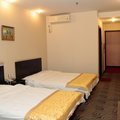#### 豪华标准间

• 不含早（预付）
• 双床
• 有窗
• 免费无线
• ￥329
• 有房
• 预付
• 预订
• 豪华标准双床间(无早)
• 双床
• 有窗
• 免费无线
• ￥331
• 有房
• 预付
• 预订
• 豪华双床间(无早)
• 双床
• 有窗
• 免费无线
• ￥331
• 有房
• 预付
• 预订
• 豪华双床间(无早)
• 双床
• 有窗
• 免费无线
• ￥331
• 有房
• 预付
• 预订
• 豪华双床间(提前1天预订)(特惠)(无早)
• 双床
• 有窗
• 免费无线
• ￥331
• 有房
• 预付
• 预订
• 标准价
• 双床
• 有窗
• 免费无线
• ￥331
• 有房
• 预付
• 预订
• (今日特价)
• 双床
• 有窗
• 免费无线
• ￥331
• 有房
• 预付
• 预订
• 标准价
• 双床
• 有窗
• 免费无线
• ￥331
• 有房
• 预付
• 预订
• 标准价
• 双床
• 有窗
• 免费无线
• ￥331
• 有房
• 预付
• 预订
• (双床)(提前1天预订)(今日特价)
• 双床
• 有窗
• 免费无线
• ￥331
• 有房
• 预付
• 预订
• (双床)(今日特价)
• 双床
• 有窗
• 免费无线
• ￥331
• 有房
• 预付
• 预订
• (双床)(特惠专享)
• 双床
• 有窗
• 免费无线
• ￥331
• 有房
• 预付
• 预订
• (提前1天预订)(特惠)
• 双床
• 有窗
• 免费无线
• ￥331
• 有房
• 预付
• 预订
• (今日特价)
• 双床
• 有窗
• 免费无线
• ￥331
• 有房
• 预付
• 预订
• (今日特价)
• 双床
• 有窗
• 免费无线
• ￥331
• 有房
• 预付
• 预订
• 标准价
• 双床
• 有窗
• 免费无线
• ￥331
• 有房
• 预付
• 预订
• 标准价
• 双床
• 有窗
• 免费无线
• ￥341
• 有房
• 预付
• 预订
• (双床)
• 双床
• 有窗
• 免费无线
• ￥347
• 有房
• 预付
• 预订
• 标准价
• 双床
• 有窗
• 免费无线
• ￥348
• 有房
• 预付
• 预订
• (双床)
• 双床
• 有窗
• 免费无线
• ￥349
• 有房
• 预付
• 预订
• (限量特价)
• 双床
• 有窗
• 免费无线
• ￥349
• 有房
• 预付
• 预订
• 标准价
• 双床
• 有窗
• 免费无线
• ￥351
• 有房
• 到店付
• 预订
• 标准价
• 双床
• 有窗
• 免费无线
• ￥352
• 有房
• 预付
• 预订
• 标准价
• 双床
• 有窗
• 免费无线
• ￥355
• 有房
• 预付
• 预订
• 标准价
• 双床
• 有窗
• 免费无线
• ￥355
• 有房
• 预付
• 预订
• 标准价
• 双床
• 有窗
• 免费无线
• ￥355
• 有房
• 预付
• 预订
• 标准价
• 双床
• 有窗
• 免费无线
• ￥355
• 有房
• 预付
• 预订
• 标准价
• 双床
• 有窗
• 免费无线
• ￥359
• 有房
• 预付
• 预订
• 不含早（预付）
• 双床
• 有窗
• 免费无线
• ￥369
• 有房
• 预付
• 预订
• (提前1天预订)
• 双床
• 有窗
• 免费无线
• ￥369
• 有房
• 预付
• 预订
• 标准价
• 双床
• 有窗
• 免费无线
• ￥369
• 有房
• 预付
• 预订
• 标准价
• 双床
• 有窗
• 免费无线
• ￥371
• 有房
• 预付
• 预订
• 标准价
• 双床
• 有窗
• 免费无线
• ￥373
• 有房
• 预付
• 预订
• (双床)
• 双床
• 有窗
• 免费无线
• ￥389
• 有房
• 预付
• 预订
• (今日特价)
• 双床
• 有窗
• 免费无线
• ￥402
• 有房
• 预付
• 预订
• (2间起订)
• 双床
• 有窗
• 免费无线
• ￥318
• 满房
• 预付
• 满房
• 豪华双床间(无早)(今日特价 )
• 双床
• 有窗
• 免费无线
• ￥331
• 满房
• 预付
• 满房
• 豪华双床间(无早)(特惠专享)(双床)
• 双床
• 有窗
• 免费无线
• ￥331
• 满房
• 预付
• 满房
• 豪华双床间(提前1天预订)(无早)(今日特价 )(双床)
• 双床
• 有窗
• 免费无线
• ￥331
• 满房
• 预付
• 满房
• 豪华双床间(度假)(今日特价 )
• 双床
• 有窗
• 免费无线
• ￥331
• 满房
• 预付
• 满房
• 豪华双床间(无早)(今日特价 )(普通,金牌,白金,钻石会员 特惠)
• 双床
• 有窗
• 免费无线
• ￥331
• 满房
• 预付
• 满房
• 豪华双床间(无早)(今日特价 )(双床)
• 双床
• 有窗
• 免费无线
• ￥331
• 满房
• 预付
• 满房
• (双床)
• 双床
• 有窗
• 免费无线
• ￥347
• 满房
• 预付
• 满房
• (2间起订)
• 双床
• 有窗
• 免费无线
• ￥347
• 满房
• 预付
• 满房
• (特惠)
• 双床
• 有窗
• 免费无线
• ￥347
• 满房
• 预付
• 满房
• 标准价
• 双床
• 有窗
• 免费无线
• ￥359
• 满房
• 预付
• 满房
• 标准价
• 双床
• 有窗
• 免费无线
• ￥359
• 满房
• 预付
• 满房
• (今日特价)
• 双床
• 有窗
• 免费无线
• ￥374
• 满房
• 预付
• 满房
• (双床)
• 双床
• 有窗
• 免费无线
• ￥423
• 满房
• 预付
• 满房#### 三人间

• 不含早（预付）
• 三张床
• 有窗
• 免费无线
• ￥384
• 2
• 预付
• 预订
• 三人间(无早)
• 三张床
• 有窗
• 免费无线
• ￥387
• 有房
• 预付
• 预订
• 标准价
• 三张床
• 有窗
• 免费无线
• ￥387
• 有房
• 预付
• 预订
• 标准价
• 三张床
• 有窗
• 免费无线
• ￥387
• 有房
• 预付
• 预订
• (今日特价)
• 三张床
• 有窗
• 免费无线
• ￥387
• 有房
• 预付
• 预订
• 标准价
• 三张床
• 有窗
• 免费无线
• ￥396
• 有房
• 预付
• 预订
• 标准价
• 三张床
• 有窗
• 免费无线
• ￥399
• 有房
• 预付
• 预订
• 标准价
• 三张床
• 有窗
• 免费无线
• ￥403
• 有房
• 预付
• 预订
• 标准价
• 三张床
• 有窗
• 免费无线
• ￥406
• 有房
• 预付
• 预订
• (特价大促销)
• 三张床
• 有窗
• 免费无线
• ￥406
• 有房
• 预付
• 预订
• 标准价
• 三张床
• 有窗
• 免费无线
• ￥408
• 有房
• 预付
• 预订
• 标准价
• 三张床
• 有窗
• 免费无线
• ￥409
• 有房
• 到店付
• 预订
• 标准价
• 三张床
• 有窗
• 免费无线
• ￥410
• 有房
• 预付
• 预订
• 标准价
• 三张床
• 有窗
• 免费无线
• ￥414
• 有房
• 预付
• 预订
• 标准价
• 三张床
• 有窗
• 免费无线
• ￥417
• 有房
• 预付
• 预订
• (限量抢购)
• 三张床
• 有窗
• 免费无线
• ￥417
• 有房
• 预付
• 预订
• (特价大促销)
• 三张床
• 有窗
• 免费无线
• ￥421
• 有房
• 预付
• 预订
• 不含早（预付）
• 三张床
• 有窗
• 免费无线
• ￥430
• 有房
• 预付
• 预订
• 标准价
• 三张床
• 有窗
• 免费无线
• ￥430
• 有房
• 预付
• 预订
• (提前1天预订)
• 三张床
• 有窗
• 免费无线
• ￥430
• 有房
• 预付
• 预订
• 标准价
• 三张床
• 有窗
• 免费无线
• ￥432
• 有房
• 预付
• 预订
• 标准价
• 三张床
• 有窗
• 免费无线
• ￥457
• 有房
• 预付
• 预订
• 标准价
• 三张床
• 有窗
• 免费无线
• ￥382
• 满房
• 预付
• 满房
• (2间起订)
• 三张床
• 有窗
• 免费无线
• ￥382
• 满房
• 预付
• 满房
• (特惠)
• 三张床
• 有窗
• 免费无线
• ￥382
• 满房
• 预付
• 满房
• 三人间(无早)(今日特价 )
• 三张床
• 有窗
• 免费无线
• ￥387
• 满房
• 预付
• 满房
• 标准价
• 三张床
• 有窗
• 免费无线
• ￥397
• 满房
• 预付
• 满房
• 标准价
• 三张床
• 有窗
• 免费无线
• ￥397
• 满房
• 预付
• 满房
• (今日特价)
• 三张床
• 有窗
• 免费无线
• ￥420
• 满房
• 预付
• 满房
• (限量促销)
• 三张床
• 有窗
• 免费无线
• ￥439
• 满房
• 预付
• 满房
• (限量特价)
• 三张床
• 有窗
• 免费无线
• ￥449
• 满房
• 预付
• 满房
• (今日特价)
• 三张床
• 有窗
• 免费无线
• ￥457
• 满房
• 预付
• 满房
• 标准价
• 三张床
• 有窗
• 免费无线
• ￥477
• 满房
• 预付
• 满房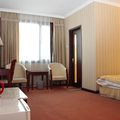#### 商务间

• 标准价
• 大床1.8米
• 有窗
• 免费无线
• ￥387
• 有房
• 预付
• 预订
• (今日特价)
• 大床1.8米
• 有窗
• 免费无线
• ￥387
• 有房
• 预付
• 预订
• (特惠专享)
• 大床1.8米
• 有窗
• 免费无线
• ￥406
• 有房
• 预付
• 预订
• 标准价
• 大床1.8米
• 有窗
• 免费无线
• ￥409
• 有房
• 到店付
• 预订
• (特惠)
• 大床1.8米
• 有窗
• 免费无线
• ￥417
• 有房
• 预付
• 预订
• (特价大促销)
• 大床1.8米
• 有窗
• 免费无线
• ￥421
• 有房
• 预付
• 预订
• (大床)
• 大床1.8米
• 有窗
• 免费无线
• ￥458
• 有房
• 预付
• 预订
• 标准价
• 大床1.8米
• 有窗
• 免费无线
• ￥382
• 满房
• 预付
• 满房
• 标准价
• 大床1.8米
• 有窗
• 免费无线
• ￥382
• 满房
• 预付
• 满房
• 家庭三人间(无早)(今日特价 )
• 大床1.8米
• 有窗
• 免费无线
• ￥387
• 满房
• 预付
• 满房
• 标准价
• 大床1.8米
• 有窗
• 免费无线
• ￥410
• 满房
• 预付
• 满房
• 标准价
• 大床1.8米
• 有窗
• 免费无线
• ￥501
• 满房
• 预付
• 满房

• 上网服务
免费wifi
• 酒店电话
010-51651678/64027118
• 停车场
未知
• 开业时间
酒店开业时间 2005-07 新近装修时间 2009-04
• 酒店设施
会议设施,无停车场,免费wifi